# J(8,2,4) Generalized Johnson Graph

The Second DIMACS Implementation Challenge: 1992-1993

Originator: Panos Pardalos

## Examples

### Basic Examples

Retrieve the graph:

 In:=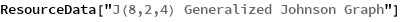Out=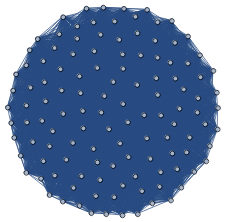Summary properties:

 In:=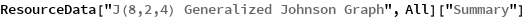Out=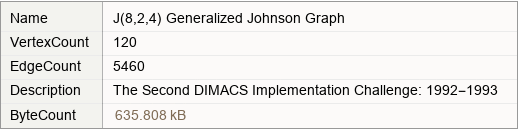### Basic Applications

Find the maximum clique:

 In:=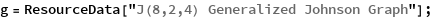In:=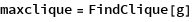Out=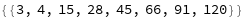In:=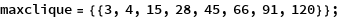Show the maximum clique:

 In:=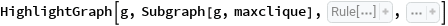Out=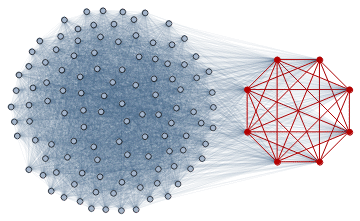Wolfram Research, "J(8,2,4) Generalized Johnson Graph" from the Wolfram Data Repository (2019)